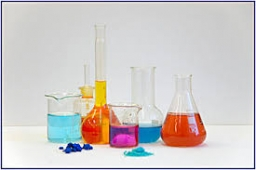# Concentration 27551

We need a 4% solution of H2O2 (hydrogen peroxide) for the disinfection solution. We only have a 40% solution available. How much water do we need to add to 100ml of the original solution to obtain the desired concentration?

x =  900 ml

### Step-by-step explanation:

0.40 · 100 = 0.04(x+100)

0.40 · 100 = 0.04·(x+100)

0.04x = 36

x = 36/0.04 = 900

x = 900

Our simple equation calculator calculates it.Did you find an error or inaccuracy? Feel free to write us. Thank you!

Tips for related online calculators
Check out our ratio calculator.
Do you have a linear equation or system of equations and looking for its solution? Or do you have a quadratic equation?